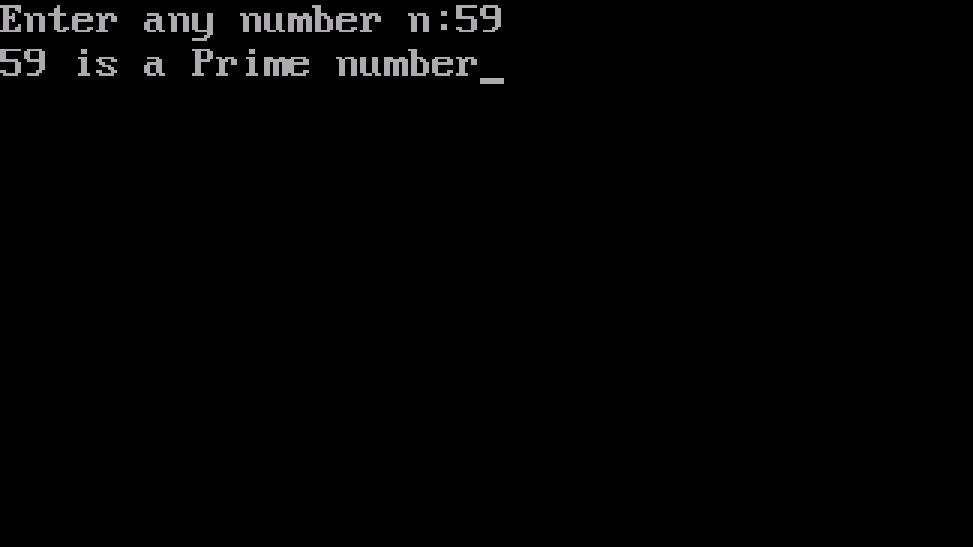# C Program To Check Prime Number

The following is a C Program to check if Given number is a Prime Number on screen after taking input from user. Let's get started.C Program To Check Prime Number

## Commands

scanf() function is used to obtain input and printf() function is used to print the string on the screen.
if() statement is used as test condition.

## Program

``````#include<stdio.h>
#include<conio.h>
void main()
{
int n,i,c=0;
clrscr();
printf("Enter any number n:");
scanf("%d",&n); /*working formula*/
for(i=1;i<=n;i++)
{
if(n%i==0)
{
c++;
}
}
if(c==2)
{
printf("%d is a Prime number",n);
}
else
{
printf("%d is not a Prime number",n);
}
getch();
}``````

## OutputOutput of Prime Number

All programs compiled in this post are compiled in AnDOSBox.

### Books I Prefer:

I hope this article was helpful to understand C Program to check if Given number is a Prime Number! Comment below, if you've got any question. Head back soon for another interesting article on C Programming.